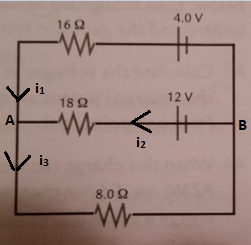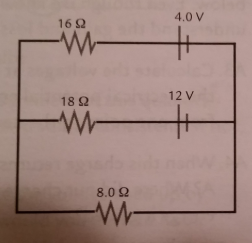# Problem: Use Kirchhoff's rules to find the current in each of the three resistors in the circuit shown. Also calculate the voltage drop across all three resistors.

###### FREE Expert SolutionUsing Kirchoff's junction rule at A:

i1 + i2 = i3

For the top loop counterclockwise:

4.0 - 16i1 + 18i2 - 12 = 0

Multiply by -1 and simplify

16i1 - 18i2 + 8 = 0  Eqn 1

For the lower loop counter-clockwise:

82% (433 ratings)###### Problem Details

Use Kirchhoff's rules to find the current in each of the three resistors in the circuit shown. Also calculate the voltage drop across all three resistors.